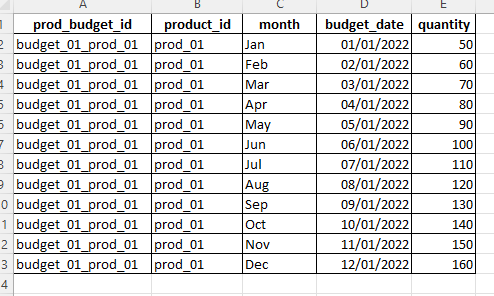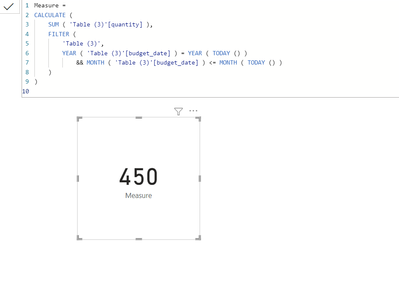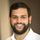cancel
Showing results for
Did you mean:Helper I

## Calculate Budget quantity till current month

Hi Guys,
I am trying to sum the quantity column from Jan to current month of this year. The measure should be dynamic that is if the month is July then the measure should calculate the quantity from Jan to July and if the month is August, then the measure should calculate quantity from Jan to August.

Thanks1 ACCEPTED SOLUTIONSuper User

Hi @powerbiss ,

You could create a measure as below:-

``````Measure =
CALCULATE (
SUM ( 'Table (3)'[quantity] ),
FILTER (
'Table (3)',
YEAR ( 'Table (3)'[budget_date] ) = YEAR ( TODAY () )
&& MONTH ( 'Table (3)'[budget_date] ) <= MONTH ( TODAY () )
)
)
``````

Output:-BR,

Samarth

Best Regards,
Samarth

If this post helps, please consider accepting it as the solution to help the other members find it more quickly.

4 REPLIES 4Super User

Hi @powerbiss ,

You could create a measure as below:-

``````Measure =
CALCULATE (
SUM ( 'Table (3)'[quantity] ),
FILTER (
'Table (3)',
YEAR ( 'Table (3)'[budget_date] ) = YEAR ( TODAY () )
&& MONTH ( 'Table (3)'[budget_date] ) <= MONTH ( TODAY () )
)
)
``````

Output:-BR,

Samarth

Best Regards,
Samarth

If this post helps, please consider accepting it as the solution to help the other members find it more quickly.Helper I

Hi Samarth,

The DAX measure worked. Thanks for your help.Super User

Try something like this

``YTD = TOTALYTD(SUM('table'[quantity]),'table'[budget_date])``Helper I

Hey Syk,

The DAX measure is showing the whole year amount.

Thanks